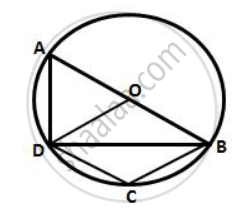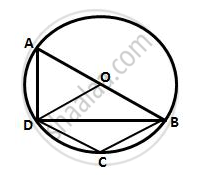Share

# In the Given Figure, Ab is a Diameter of the Circle with Centre O. Do is Parallel to Cb and ∠Dcb = 120°. Calculate: ∠Dab, Also Show that the δAod is an Equilateral - Mathematics

Course

#### Question

In the given figure, AB is a diameter of the circle with centre O. DO is parallel to CB and ∠DCB = 120°.
Calculate :  ∠DAB
Also show that the ΔAOD is an equilateral triangle .#### Solution∴ ∠DCB + ∠DAB = 180°

(pair of opposite angles in a cyclic quadrilateral are supplementary)

⇒ ∠DAB =180° -120° = 60°

Is there an error in this question or solution?